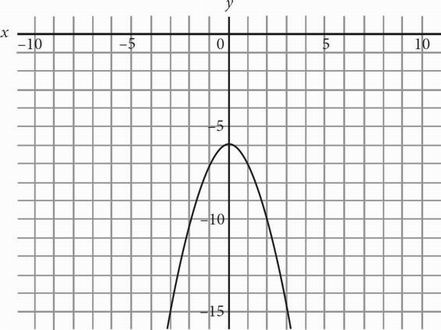# SAT Math Multiple Choice Question 156: Answer and Explanation

### Test Information

Question: 156

6.Which of the following equations best describes the figure above?

• A. y = -x4 + 6
• B. y = -(x2 + 6)
• C. y = -x2 + 6
• D. y = x4 + 6